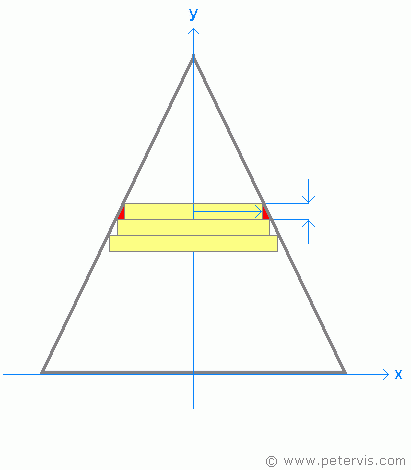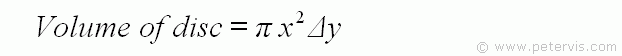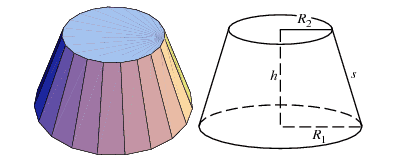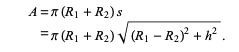# Surface of a cone by integration

themagiciant95
If i want to calculate the volume of a cone i can integrate infinitesimal disks on the height h of the cone.I was told that if i want to calculate the surface of the cone, this approximation is not correct and i have to take the slanting into account, this means that instead of infinitesimal disk i have to consider infinitesimal conical frustum and integrate the lateral surface of each infinitesimal conical frustum. Why for the surface i can't use infinitesimal disks as for the volume calculation ?Furthermore, the formula for the lateral surface of a conical frustum is :Now, let's consider an infinitesimal conical frustum.
For $h\rightarrow 0$ , $R_{1}\approx R_{2}$ so $(R_{1} - R_{2})^{2} \rightarrow 0$ and the surface of an infinitesimal conical frustum would be$2\pi R_{2}\,dh$, however i was told that it's not correct. The correct one is $2\pi R_{2}\sqrt{ d(R_{2}-R_{1})^{2}+ dh^2}$, why?

mitochan
As for surfaces of full cone
The bottom circle pf radius r:
$$A_b=\pi r^2$$
The side partial circle for cone height h, so with central angle ##\phi##
$$A_s=\pi (r^2+h^2) \frac{\phi}{2\pi}$$
the bottom circle and the side partial circle share the periphery
$$2\pi r = \phi \sqrt{r^2+h^2}$$
$$\phi=\frac{2\pi r}{\sqrt{r^2+h^2}}$$
So
$$A_s=\pi r \sqrt{r^2+h^2}$$
From this fulll cone case you can deduce the answer for your case of cut parts.

For h→0h\rightarrow 0 , R1≈R2R_{1}\approx R_{2} so (R1−R2)2→0(R_{1} - R_{2})^{2} \rightarrow 0 and the surface of an infinitesimal conical frustum would be2πR2dh2\pi R_{2}\,dh, however i was told that it's not correct. The correct one is 2πR2√d(R2−R1)2+dh22\pi R_{2}\sqrt{ d(R_{2}-R_{1})^{2}+ dh^2}, why?

The square of infinitesimal appears in square root. So it should be treated not second but first degree infinitesimal in the formula.

Last edited:
•PeroK and sysprog
Homework Helper
Gold Member
2022 Award
If i want to calculate the volume of a cone i can integrate infinitesimal disks on the height h of the cone.

View attachment 259410

View attachment 259413
View attachment 259414

I was told that if i want to calculate the surface of the cone, this approximation is not correct and i have to take the slanting into account, this means that instead of infinitesimal disk i have to consider infinitesimal conical frustum and integrate the lateral surface of each infinitesimal conical frustum. Why for the surface i can't use infinitesimal disks as for the volume calculation ?

View attachment 259411

Furthermore, the formula for the lateral surface of a conical frustum is :

View attachment 259412

Now, let's consider an infinitesimal conical frustum.
For $h\rightarrow 0$ , $R_{1}\approx R_{2}$ so $(R_{1} - R_{2})^{2} \rightarrow 0$ and the surface of an infinitesimal conical frustum would be$2\pi R_{2}\,dh$, however i was told that it's not correct. The correct one is $2\pi R_{2}\sqrt{ d(R_{2}-R_{1})^{2}+ dh^2}$, why?

It's an interesting question of how you know when these estimates are correct. Let's look at the two cases of the volume and the area. If we look at the volume, we can see that:
$$\pi R_1^2 h < V < \pi R_2^2 h$$
If we now imagine an infinitesimal frustrum of height ##dh##, we see that:
$$\pi r^2 dh < dV < \pi (r + dr)^2 dh = \pi (r^2 +2rdr + dr^2)dh$$
And, as we can neglect higher order terms, this gives us a valid equation for the infinitesimal volume element:
$$dV = \pi r^2 dh$$
When we look at the area, however, we see that:
$$2\pi R_2 s < A < 2\pi R_1 s$$
And, for an infinitesimal frustrum:
$$2\pi r s < A < 2\pi (r+dr)s$$
And, we can see that:
$$s = \frac l h dh$$
Where ##l## is the slant height of the full cone.
This gives us the equation for the infinitesimal surface area element:
$$dA = 2\pi r \frac l h dh = \frac{2\pi l}{R}rdr$$
The moral is that you really have to ensure that your estimates are valid to the first order in the infinitesimals.

Last edited:
mitochan
Now, let's consider an infinitesimal conical frustum.
For h→0h\rightarrow 0 , R1≈R2R_{1}\approx R_{2} so (R1−R2)2→0(R_{1} - R_{2})^{2} \rightarrow 0 and the surface of an infinitesimal conical frustum would be2πR2dh2\pi R_{2}\,dh, however i was told that it's not correct. The correct one is 2πR2√d(R2−R1)2+dh22\pi R_{2}\sqrt{ d(R_{2}-R_{1})^{2}+ dh^2}, why?

$$d(R_2-R_1)^2=[d(R_2-R_1)]^2 =(dR_2-dR_1)^2 \neq d[(R_2-R_1)^2]=2(R_2-R_1)(dR_2-dR_1)$$
might be of your help. RHS is first order and LHS is second order infinitesimals.
You do not have to refer the estimation
$$(R_2-R_1)^2 \rightarrow 0$$
anywhere.

Homework Helper
Gold Member
2022 Award
$$d(R_2-R_1)^2=[d(R_2-R_1)]^2 =(dR_2-dR_1)^2 \neq d[(R_2-R_1)^2]=2(R_2-R_1)(dR_2-dR_1)$$
might be of your help. RHS is first order and LHS is second order infinitesimals.
You do not have to refer the estimation
$$(R_2-R_1)^2 \rightarrow 0$$
anywhere.

For the frustrum, you can do:
$$dA = 2\pi r dl$$
And, with ##L## as the slant length of the fulcrum, we have:
$$dl = \frac{L}{R_2 - R_1}dr$$
This gives:
$$A = \int_{R_1}^{R_2} \frac{2\pi L}{R_2 - R_1} r dr = \frac{\pi L}{R_2 - R_1}(R_2^2 - R_1^2) = \pi L (R_1 + R_2)$$
And, in terms of ##h##:
$$A = \pi (R_1 + R_2)L = \pi(R_1 + R_2)\sqrt{(R_2-R_1)^2 + h^2}$$
Finally, this makes sense as:
$$A = 2 \pi R_{avg} L$$

themagiciant95
It's an interesting question of how you know when these estimates are correct. Let's look at the two cases of the volume and the area. If we look at the volume, we can see that:
$$\pi R_1^2 h < V < \pi R_2^2 h$$
If we now imagine an infinitesimal frustrum of height ##dh##, we see that:
$$\pi r^2 dh < dV < \pi (r + dr)^2 dh = \pi (r^2 +2rdr + dr^2)dh$$
And, as we can neglect higher order terms, this gives us a valid equation for the infinitesimal volume element:
$$dV = \pi r^2 dh$$
When we look at the area, however, we see that:
$$2\pi R_2 s < A < 2\pi R_1 s$$
And, for an infinitesimal frustrum:
$$2\pi r s < A < 2\pi (r+dr)s$$
And, we can see that:
$$s = \frac l h dh$$
Where ##l## is the slant height of the full cone.
This gives us the equation for the infinitesimal surface area element:
$$dA = 2\pi r \frac l h dh = \frac{2\pi l}{R}rdr$$
The moral is that you really have to ensure that your estimates are valid to the first order in the infinitesimals.

Why can we neglect those higher-order terms ? Furthemore, if we do this than $\pi r^2 dh < dV < \pi (r + dr)^2 dh = \pi (r^2 +2rdr + dr^2)dh$ becomes pointless
ps: i think i have not understood why you have underlined this chain of disequalities

Homework Helper
Gold Member
2022 Award
Why can we neglect those higher-order terms ? Furthemore, if we do this than $\pi r^2 dh < dV < \pi (r + dr)^2 dh = \pi (r^2 +2rdr + dr^2)dh$ becomes pointless

Neglecting higher order terms is precisely what I've done. In your OP you also neglected terms that were of first order - in your infinitesimal equation for the area element.

themagiciant95
Yes but i don't understand the rules when dealing with infinitesimal quantities. It's the only thing i can't deeply understand in undergraduate physics and i haven't found a book about it.

ps: i think i have not understood why you have took care of this chain of disequalities: $\pi r^2 dh < dV < \pi (r + dr)^2 dh = \pi (r^2 +2rdr + dr^2)dh$ . For what purpose ?

Homework Helper
Gold Member
2022 Award
Yes but i don't understand the rules when dealing with infinitesimal quantities. It's the only thing i can't deeply understand in ungraduate physics and i haven't found a book about it.

ps: i think i have not understood why you have took care of this chain of disequalities: $\pi r^2 dh < dV < \pi (r + dr)^2 dh = \pi (r^2 +2rdr + dr^2)dh$ . For what purpose ?

That was to check that the equation ##dV = \pi r^2 dh## is valid to first order.

Whereas, you have:

the surface of an infinitesimal conical frustum would be $2\pi R_{2}\,dh$,

But, the width of the area element is ##ds##, which is not equal to ##dh## to first order. Instead, we have:
$$ds = \frac L R dr$$
Where ##L## and ##R## are the slant height and maximum radius of the full cone.